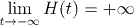Analysis WebNotes
arrow_back arrow_forward

# Class Contents

## Variations on the Theme of Limits

There are numerous variants of limits of functions that we can introduce. We can look at the limit of a function f(x) as x goes to positive infinity, or as x goes to negative infinity. The limit itself might be a real number, or the function itself might converge to positive or negative infinity. We can also look at the limit of f(x) as x approaches a from just one side, the left or the right. Again, this limit might be a real number or it might be plus or minus infinity.

What we don't want to do, is list all possible definitions of this kind of limit (I count 15 of them). The key is understanding what are the ingedients that go into different kinds of limit, and how to "mix 'n' match" these ingredients to make any limit definition you need.

The first section here looks at limits as x approaches infinity, and the second section deals with left and right limits. There isn't very much on limits where the function goes to plus or minus infinity, but there is an example in Example 2.

This page emphasizes asking questions, and you should make an effort to try to write down the correct definitions yourself, before clicking to see the answers. There are a few extra questions at the end of the page, to test your understanding.

## Limits at Infinity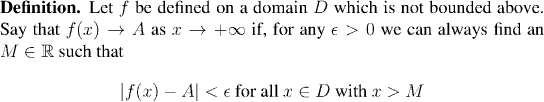Remark:

Note that the condition "D is not bounded above" is equivalent to saying "there is a sequence in D which converges to positive infinity". Thus this condition serves the same role in this definition as requiring that a be a limit point of D did in the original definition of limit.

Question: What do you think is the definition of the limit as x goes to minus infinity of f(x)?

Example: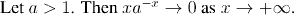## Left and Right Limits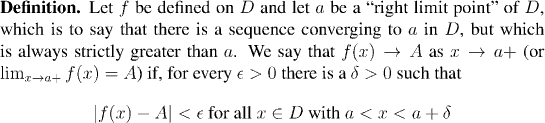Question: What do you think is the definition of the left limit of a function?

Example: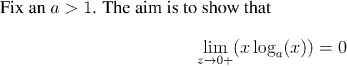## Some Questions

Question: What would you need to do, to show that the limit as x approaches a of f(x) is plus infinity?

Question: What is the definition of